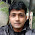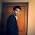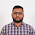# R : Create Sample / Dummy Data

This tutorial explains how to create sample / dummy data. It is very useful to know how we can build sample data to practice R exercises. 'Sample/ Dummy data' refers to dataset containing random numeric or string values which are produced to solve some data manipulation tasks. For example, you want to learn how to apply logical conditions (IF ELSE) in R. To gain practical experience, it is important to practice it with sample datasets.

Method 1 : Enter Data Manually

The simplest method is to type data values in R editor and submit it. See the example below. The program below creates 3 variables - ID, var1 and var2. The name of data frame would be df1.
df1 <- data.frame(ID = c(1, 2, 3, 4, 5),
var1 = c('a', 'b', 'c', 'd', 'e'),
var2 = c(1, 1, 0, 0, 1))Sample Data in R
Note : Since var1 is a character variable, it is entered in a single quote.

Method 2 : Sequence of numbers, letters, months and random numbers
1. seq(1, 16, by=2) - sequence of numbers from 1 to 16 increment by 2.
2. LETTERS[1:8] - the 8 upper-case letters of the english alphabet.
3. month.abb[1:8] - the three-letter abbreviations for the first 8 English months
4. sample(10:20, 8, replace = TRUE) - 8 random numbers with replacement from 10 to 20.
5. letters[1:8] - the 8 lower-case letters of the english alphabet.
df2 <- data.frame(a = seq(1,16,by=2), b = LETTERS[1:8], x= month.abb[1:8], y = sample(10:20,8, replace = TRUE), z=letters[1:8])Sequence / Random Values in R

Method 3 : Create numeric grouping variable
df3 = data.frame(X = sample(1:3, 15, replace = TRUE))
It returns 15 random values with replacement from 1 to 3.

Method 4 : Random Numbers with mean 0 and std. dev 1
set.seed(1)
df4 <- data.frame(Y = rnorm(15), Z = ceiling(rnorm(15)))

Method 5 : Create binary variable (0/1)
set.seed(1)
ifelse(sign(rnorm(15))==-1,0,1)
In the code above, if sign of a random number is negative, it returns 0. Otherwise, 1.

Method 6: Copy Data from Excel to R

Method 7: Create character grouping variable
mydata = sample(LETTERS[1:5],16,replace = TRUE)
It returns random 16 characters having alphabets ranging from "A" to "E".Related PostsDeepanshu founded ListenData with a simple objective - Make analytics easy to understand and follow. He has over 8 years of experience in data science. During his tenure, he has worked with global clients in various domains like Banking, Insurance, Telecom and Human Resource.

11 Responses to "R : Create Sample / Dummy Data"
1.Hi ,
Could you please tell me what's exactly happening in "Create binary variable (0/1):" I could understand the syntax

1.If sign of a random number is negative, it returns 0. Otherwise, 1.

2.2.hi
fine thanks

3.Nice Work Deepanshu, your tutorial are very good short and crisp.

4.Bhalla saab... Bas maja aa gaya. I have actually bookmarked it as one of my favorites.

5.hi ,could you please tell me in method 4 why we use 15 in generating random number

1.15 is the number of random variables that you want you can fill any number in that

6.Nice information...

7.Wonderful! tutorial!!

8.Hi
Could you please tell me about binary conditions that can we create it with random numbers only?

Next → ← Prev
Love this Post? Spread the Word!
Share